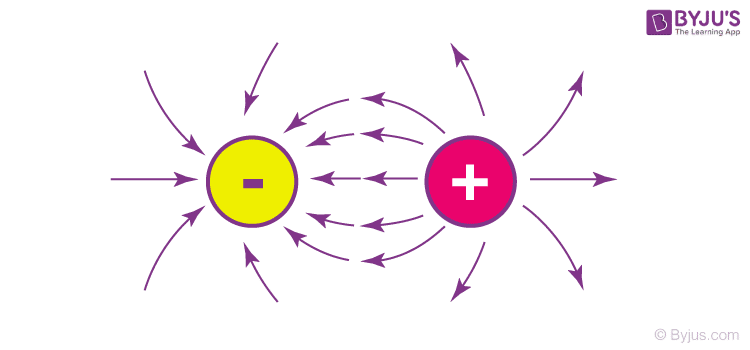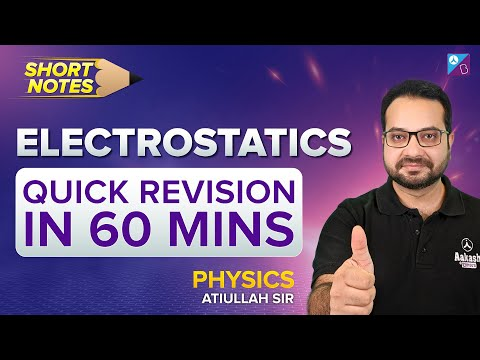Defend yourself better by mastering the science of immunity and vaccines. Secure your free spot, now! Defend yourself better by mastering the science of immunity and vaccines. Secure your free spot, now!

# Electrostatics

Electrostatics is the branch of physics that studies the charges at rest. In this article, you will explore electrostatics in detail.

## What is Electrostatics?

Electrostatics is a branch of physics that deals with the phenomena and properties of stationary or slow-moving electric charges. Electrostatic phenomena arise from the forces that electric charges exert on each other and are described by Coulomb’s law. Even though electrostatically induced forces seem to be relatively weak.## Coulomb’s Law of Electrostatics

We begin with the magnitude of the electrostatic force between two point charges q  and Q . It is convenient to label one of these charges, q , as a test charge, and call Q a source charge. As we develop the theory, more source charges will be added. If r  is the distance between two charges, then the force of electrostatic formula is:

$$\begin{array}{l}\large F=\frac{1}{4 \pi \xi _{0}}\frac{qQ}{r^{2}}=k_{e}\frac{qQ}{r^{2}}\end{array}$$

Or

$$\begin{array}{l}\large F=k\frac{q_{1}q_{2}}{d^{2}}\end{array}$$

Here is a rapid-fire quiz on Electrostatics between three students! We host such Rapid Fire quizzes every Monday!## Electric field

Electric field lines help visualize the electric field. Field lines begin on a positive charge and terminate on a negative charge. Electric field lines are parallel to the direction of the electric field, and the density of these field lines is a measure of the magnitude of the electric field at any given point.

We show charge with “q” or “Q,” and the smallest unit charge is 1.6021 x 10-19 Coulomb (C). One electron and a proton have the same amount of charge.

### Positively Charged Particles

In these particles, the numbers of positive ions are larger than the numbers of negative ions. This means the numbers of protons are larger than the number of electrons. To neutralize positively charged particles, electrons from the surroundings come to this particle until the number of protons and electrons becomes equal.

### Negatively Charged Particles

Similarly numbers of electrons are larger than the number of protons. To neutralize negatively charged particles, since protons cannot move and cannot come to negatively charged particles, electrons move to the ground or any other particle around.

### Neutral Particles

Neutral particles include equal numbers of protons and electrons. They have both protons, neutrons and electrons; however, the numbers of positive ions equal the numbers of negative ions.

## Electrostatics Examples

There are many examples of electrostatic phenomena:

• The attraction of the plastic wrap to your hand after you remove it from a package.
• The attraction of paper to a charged scale.
• The apparently spontaneous explosion of grain silos.
• The damage of electronic components during manufacturing.
• Photocopier and laser printer operation.

## Electrostatics Questions

Q1. Two equal and like charges are placed at a distance d = 6 cm. They exert a force 12 × 10-3 N on each other. What is the magnitude of each charge?

Solution:

The magnitude of force between two static charges separated by a distance ‘d’ is given by Coulomb’s equation as follows:

$$\begin{array}{l}F=k\frac{\left | q_{1}q_{2} \right |}{d^2}\end{array}$$

k is Coulomb’s constant and has a value 8.99 x 109 N.m2/C2

Let the magnitude of charges be |q1| = |q2| = |q|.

Substituting the values in the equation, we get

$$\begin{array}{l}12\times 10^{-3}=(8.99\times 10^{9})\times \frac{q^{2}}{(0.06)^2}\end{array}$$

$$\begin{array}{l}q^2=48\times 10^{-16}\end{array}$$

$$\begin{array}{l}q=6.9\times 10^{-8}\,C\end{array}$$

The magnitude of each charge is 6.9 × 10-8 C.

## Suggested Videos for Electrostatics

### Coulomb’s Law Explained### Gauss Law Explained### Vector Form of Coulomb’s Law### Electrostatics Solved Questions## Frequently Asked Questions – FAQs

Q1

### What is electrostatics?

Electrostatics is a branch of physics that deals with the study of stationary electric charges.
Q2

### How does electrostatics work?

Electrostatic phenomena arise from the forces that electric charges exert on each other and are described by Coulomb’s law. Even though electrostatically induced forces seem to be relatively weak.
Q3

### Why is electrostatic force conservative?

The electrostatic force is conservative in nature because the work done on the charge is independent of the path taken.
Q4

### What are the examples of electrostatics?

The attraction of the plastic wrap to your hand after you remove it from a package and the attraction of paper to a charged scale.
Q5

### Why is electrostatic force a central force?

The electrostatic force is called a central force because it acts along the line joining two charges.

## Overview of Electrostatics

#### Properties of Charge & Coulomb’s Law#### Superposition Principle & Electric Field Lines#### Electric Field For Extended Bodies#### Electric Potential inside a Metal Body#### Electric Dipole Moment Inside a Metal Body#### Electric Flux & Gauss Law#### Electric Potential Due to Charged Body#### Electric Charges, Field and PotentialStay tuned to BYJU’S and Fall in Love with Learning!